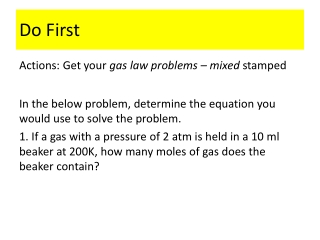DownloadDownload PresentationDo First

# Do First

Télécharger la présentation## Do First

- - - - - - - - - - - - - - - - - - - - - - - - - - - E N D - - - - - - - - - - - - - - - - - - - - - - - - - - -
##### Presentation Transcript

1. Do First Actions: Get your gas law problems – mixed stamped In the below problem, determine the equation you would use to solve the problem. 1. If a gas with a pressure of 2 atm is held in a 10 ml beaker at 200K, how many moles of gas does the beaker contain?

2. Dalton’s Law of Partial Pressures

3. Dalton’s Law of Partial Pressures • States that the total pressure of mixture of gases is equal to the sum of the partial pressure of the individual gases • Partial pressure is the pressure of each gas in a mixture Ptotal = P1 + P2 + P3 + …

4. Gas Collected by Water Displacement • http://highered.mheducation.com/olcweb/cgi/pluginpop.cgi?it=swf::100%::100%::/sites/dl/free/0072512644/117354/04_Collecting_a_Gas_Over_Water.swf::Collecting%20a%20Gas%20Over%20Water

5. Gas Collected Over Water • In the lab, gas produced by a reaction can be collected and measured by water displacement • When a gas is collected over water, what is collected is a mixture of the gas produced PLUS water vapor due to it evaporating • The total pressure in the collection tube is due to the partial pressure of the gas collected and the partial pressure of the water vapor. Ptotal= PGas + PH2O

6. Gas Collected Over Water • When finding the pressure of a gas collected over water, WE DON’T WANT TO INCLUDE THE PRESSURE OF THE WATER VAPOR. • We can find the pressure of water vapor by LOOKING AT A DATA TABLE because the water vapor pressure depends only on the temperature, NOT how big the container is or the pressure of the other gas

7. Gas Collected Over Water

8. Practice 1. A gas is collected by water displacement at 50oC and a barometric pressure of 712.6 mmHg. What is the pressure exerted by the “dry” gas (without water)?

9. Practice 4. Nitrogen, oxygen, and carbon dioxide are in a closed container. The oxygen has a partial pressure of 15.5 mm Hg. The nitrogen has a partial pressure of 45.8 mm Hg. The total pressure of all gases is 83.4 mm Hg. What is the partial pressure of the carbon dioxide?

10. Practice 5. A gas is collected by water displacement so that its partial pressure is 50.00 kPa. The total pressure of the gas over water is 75.02 kPa. • What is the partial pressure of water? • What is the temperature at which the gas was collected?

11. DYL • 1. The total pressure is equal to __________. • 2. If a beaker contains oxygen and nitrogen gas, what is the pressure of the oxygen gas if the total pressure in the beaker is 20 atm and the pressure of nitrogen is 15 atm?